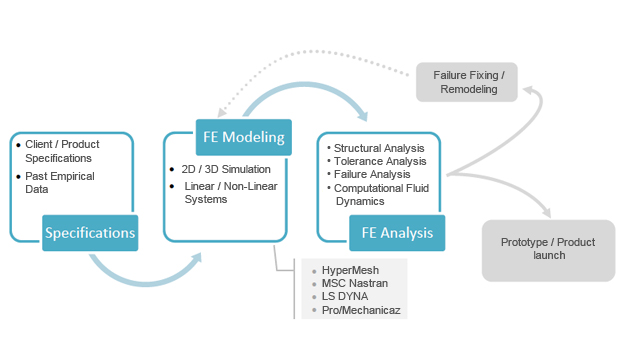### Predictive Engineering

Predictive modeling is the process of creating, testing and validating a model to best predict the probability of an outcome. A number of modeling methods from machine learning, artificial intelligence, and statistics.Predictive modeling is a commonly used statistical technique to predict future behavior. Predictive modeling solutions are a form of data-mining technology that works by analyzing historical and current data and generating a model to help predict future outcomes. In predictive modeling, data is collected, a statistical model is formulated, predictions are made, and the model is validated (or revised) as additional data becomes available.